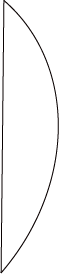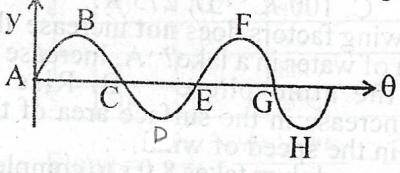## Physics 2018 Past Question | WAEC

Study the following past questions and answers for JAMBWAEC  NECO and Post-JAMB. Get prepared with official past questions and answers for upcoming examinations.

21. Rainbow formation is a natural phenomenon illustrating that white light

• A. splits into three components after rainfall
• B. is dispersed by glass prisms
• C. splits into its constituent colours before rainfall
• D. is dispersed into its constituent colours after rainfall

22.The lens illustrated above can best be described as a

• A. converging meniscus
• B. plano-convex
• C. diverging meniscus
• D. bi-convex

23. Two plane mirrors are inclined to each other such that an object placed between them has 11 images. Determine the angle of inclination

• A. 30°
• B. 45°
• C. 60°
• D. 90°

24. The pitch of a note is not affected by

• A. frequency
• B. tension in a vibrating string
• C. mass of a vibrating string
• D. amplitude of sound wave

25. When two flat plate conductors are arranged in such a way that they possess equal and opposite charges seperated by a dielectric, they form a/an

• A. electroscope
• B. electrophorus
• C. capacitor
• D. cell

26.Which electrical device is illustrated above?

• A. a.c. generator
• B. a.c. motor
• C. d.c. generator
• D. d.c. motor

27. A simple barometer is constructed using a liquid Q1 of density 16.2g/c. Calculate the least lenth of the tube to be used to measure pressure of 1.5atm.  [Density of mercury = 13.6g/c, 1atm = 76.0 cm of Mercury]

• A. 1358mm
• B. 957mm
• C. 760mm
• D. 638mm

28.The diagram above illustrates the mercury-in-glass thermometer used for determining the temperature of a room. Use the data on it to determine the room temperature.

• A. 3.3°C
• B. 7.0°C
• C. 13°C
• D. 30.0°C

29. To obtain a virtual, erect and magnified image from a thin converging lens, an object must be placed

• A. between the focus and the lens
• B. at the focus
• C. between the focal length and twice the focal length
• D. beyond twice the focal length

30.The diagram above represents a transverse travelling wave. Which two points are 180° out of phase?

• A. A and E
• B. B and D
• C. C and F
• D. D and H

## All Subjects

Subscribe
Notify ofInline Feedbacks